# Reason + factorial - math problems

#### Number of problems found: 12

• Big factorial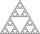How many zeros end number 116! ?
• Bookshelf and books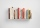How many ways can we place 7 books in a bookshelf?
• Seating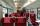How many ways can 6 people sit on 6 numbered chairs (e. g. , seat reservation on the train)?
• Combinations of sweatersI have 4 sweaters two are white, 1 red and 1 green. How many ways can this done?
• LunchSeven classmates go every day for lunch. If they always come to the front in a different order, will be enough school year to take of all the possibilities?
• Combi-triangle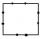On each side of the square is marked 10 different points outside the vertices of the square. How many triangles can be constructed from this set of points, where each vertex of the triangle lie on the other side of the square?
• Cinema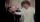How many ways can be divided 11 free tickets to the premiere of "Jáchyme throw it in the machine" between 6 pensioners?
• Math logicThere are 20 children in the group, each two children have a different name. Alena and John are among them. How many ways can we choose 8 children to be among the selected A) was John B) was John and Alena C) at least one was Alena, John D) maximum one wa
• Cups on the shelf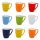2 green, 3 red and 2 yellow cups should be placed side by side on the shelf. a) How many different ways of setting up can arise? b) How many different ways of arranging can arise if cups of the same color stand side by side?
• Five letters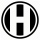How many ways can five letters be arranged?
• Desks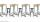A class has 20 students. The classroom consists of 20 desks, with 4 desks in each of 5 different rows. Amy, Bob, Chloe, and David are all friends, and would like to sit in the same row. How many possible seating arrangements are there such that Amy, Bob,
• Wagons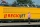We have six wagons, two white, two blue, and two red. We assemble trains from them, wagons of the same color are exactly the same, so if we change only two white wagons on a train, it's still the same train, because I don't know any different. How many di

We apologize, but in this category are not a lot of examples.
Do you have an interesting mathematical word problem that you can't solve it? Submit a math problem, and we can try to solve it.

We will send a solution to your e-mail address. Solved examples are also published here. Please enter the e-mail correctly and check whether you don't have a full mailbox.

Please do not submit problems from current active competitions such as Mathematical Olympiad, correspondence seminars etc...

Reason - math word problems. Factorial - math word problems.